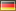OpenClonk Forum
Topic General / Help and Questions / Sorting proplists by specific key valueBy Pyrit Date 2018-04-25 22:04I want to sort all proplists inside one master proplist by a specific key. The Key is "x" in this case. An example of what I am trying:

`this.master_proplist ={    a =    {        x = 1,    },    b =    {        x = 4,    },    c =    {        not_x = 3,    },    deeper =    {        d =        {             x = 2,        },    },}`

In this case I would like to get something like:
`sorted_array = [master_proplist.a, master_proplist.deeper.d, master_proplist.b]`

So, when I want to add something to the proplist with the lowest x, I could do:
`sorted_array.additional_stuff = 42`

The result would be:
`this.master_proplist ={    a =    {        x = 1,        additional_stuff = 42,    },    b =    {        x = 4,    },    c =    {        not_x = 3,    },    deeper =    {        d =        {             x = 2,        },    },}`

Unfortunately, I could not come up with a solution for this. I have tried with GetProperties(), but I could not get into the deeper levels of the proplist. I also get back only an array of strings... Is it even possible to get a result in the form of a pointer, like `master_proplist.a`, to use at a later point in the script?

Or is there any other solution for setting a value in a sub-proplist that contains a specific key?By Luchs Date 2018-04-25 23:39 Edited 2018-04-25 23:43So the actual question here is how to find all possibly nested proplists with a certain property? Here's a function to do that:

`func FindProplistsWithProperty(proplist p, string key){  var result = [];  for (var k in GetProperties(p))  {    var v = p[k];    if (k == key)      result[GetLength(result)] = p;    if (GetType(v) == C4V_PropList)      result[GetLength(result):] = FindProplistsWithProperty(v, key);  }  return result;}`

Combined with `SortArrayByProperty()`, I think it does what you want:

`func Main() {  var master_proplist =  {    a =    {      x = 1,    },    b =    {      x = 4,    },    c =    {      not_x = 3,    },    deeper =    {      d =      {        x = 2,      },    },  };  var sorted_array = FindProplistsWithProperty(master_proplist, "x");  SortArrayByProperty(sorted_array, "x");  Log("%v", sorted_array); // result: [{x = 1}, {x = 2}, {x = 4}]  sorted_array.additional_stuff = 42;  Log("%v", master_proplist); // result: {a = {additional_stuff = 42, x = 1}, b = {x = 4}, c = {not_x = 3}, deeper = {d = {x = 2}}}}`By Clonkonaut Date 2018-04-25 23:43Damn. I also came up with a solution. But I guess it's the same. :(

`global func PyritsSecretProplistSorter(proplist list, string key_to_sort_by){  var sorted_array = [];  // Get all properties of the list  var properties = GetProperties(list);  // Cycle through the proplist and check for sub-proplists  for (var property in properties)  {    if (GetType(list[property]) != C4V_PropList)      continue;    // Push to array if the required property is present    if (list[property][key_to_sort_by] != nil)      PushBack(sorted_array, list[property]);    // Do extremely dangerous recursion call    var to_add = PyritsSecretProplistSorter(list[property], key_to_sort_by);    if (to_add != nil && GetLength(to_add) > 0)      sorted_array = Concatenate(sorted_array, to_add);  }  // And now we can sort everything!  SortArrayByProperty(sorted_array, key_to_sort_by);  return sorted_array;}global func TestPyrit(){  var weird_proplist = {    a =    {      x = 1,    },    b =    {      x = 4,    },    c =    {      not_x = 3,    },    deeper =    {      d =      {         x = 2,      },    }  };  var awesome_result = PyritsSecretProplistSorter(weird_proplist, "x");  Log("%v", weird_proplist);  Log("%v", awesome_result);  // Additional stuff  awesome_result.additional_stuff = 42;  Log("%v", weird_proplist);}`

{a = {x = 1}, b = {x = 4}, c = {not_x = 3}, deeper = {d = {x = 2}}}
[{x = 1}, {x = 2}, {x = 4}]
{a = {x = 1}, b = {x = 4}, c = {not_x = 3}, deeper = {d = {additional_stuff = 42, x = 2}}}
-> Scenario::TestPyrit()By Caesar Date 2018-04-26 00:29
Now benchmark them against each other.

[Edit:] Both do crash with cyclic proplists, right?True. My solution only sorts once and doesn't copy arrays as much!It would probably not crash but stop and show an error about too much recursion.By ZapperDate 2018-04-26 11:19What are you trying to do?I am trying to come up with a solution for controlling menus with keyboard.
My attempt is to somehow retrieve the coordinates of selectable windows. So you can jump to the window with the next higher x coordinate when pressing right, e.g.
Topic General / Help and Questions / Sorting proplists by specific key value